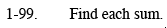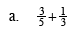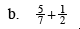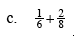### Home > MC2 > Chapter 1 > Lesson 1.2.4 > Problem1-99

1-99.Find a common denominator.

$\frac{9}{15} + \frac{5}{15} = \frac{14}{15}$

$\text{By multiplying the first fraction by } \frac{3}{3} \text{ and the second by } \frac{5}{5} \text{ you will achieve a common denominator of 15.}$

Now, you should be able to add the numerators together to get the correct sum.See part (a).See part (a).